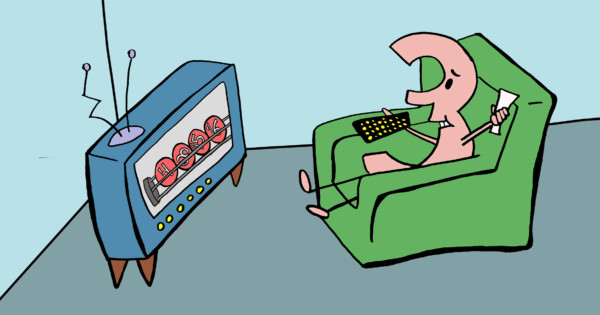# Word Problem Wednesday: Probability and the Lottery!

By Damaris | Added Jan 13, 2016It's another Word Problem Wednesday! Today's word problem explores probability as it relates to the lottery. Give it a shot—we'll update this article with the answer tomorrow!

For a certain lottery, there are 20 balls numbered 1 to 20. You select 4 numbers. Lottery officials then draw 4 balls without replacement. You win if you match the 4 selected numbers. What is the probability of winning this lottery?

Update: Here's the solution!

There are 4 winning numbers to select for the first drawing and 20 numbers to choose from, so the probability that the first number drawn is a winning number is 4/20.

For the second drawing, there are now 3 winning numbers to select for the second drawing and 19 numbers to choose from, so the probability that the second number drawn is a winning number is 3/19.

Continuing this pattern, the probability that the third drawing is a winning number is 2/18, and the probability that the fourth drawing is a winning number is 1/17.

Multiplying these fractions together gets us 4/20 x 3/19 x 2/18 x 1/17 = 1/4,845.

Alternatively, since order does not matter, this is a combination. From the 20 total balls, we are selecting 4 of them. 20C4 = 20! / [(20 – 4)! x 4!] = 4,845, so there is 4,845 different combinations of drawing 4 balls from 20 total balls. Since there is only 1 winning combination, the probability of selecting the winning combination is 1/4,845.